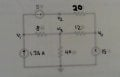# Need help with this circuit

#### kirit

Joined Sep 9, 2008
35
Hi, need help determining v1,v2,v3,v4. My answer isnt the same as another student and i need to know who is right.
here is the circuit:Ok, this is what I get:
v1=27.0248V (node A)
v2=22.0248V (node B)
v3=19.8347V (node D)
v4=15.0000V (node C)
The other person is getting answer close but not exactly the same.
Here is what equations im getting:

Supernode AB: (Vb-Vc)/20+(Va-Vd)/8=1.25 simplifies to 7Vb -5Vd=55
Node D: Vd/40 + (Vd-Vc)/12 + (Vd-Va)/8=0 simplifies to 28Vd - 15Vb = 225
Knowns:
Va-Vb=5
Vc=15

#### hitmen

Joined Sep 21, 2008
159

Va = 2710/121 = 22.36969
Vb= Va +5
Vc= 2100/121 = 17.355
Vd = 15 (obvious)

Do check my answers I could be wrong.
Do reply if I got it wrong.

#### kirit

Joined Sep 9, 2008
35
looks like your getting answers closer (almost exactly) to his than mine. Looking at my equation can you spot my error in thinking/execution?

#### hitmen

Joined Sep 21, 2008
159
I think there is something wrong with your simplification. I can't pinpoint where it is.
But there should be an error in 7Vb -5Vd=55 & 28Vd - 15Vb = 225

#### hitmen

Joined Sep 21, 2008
159
I made 1 error Va should have a negative sign. I got the same results using mesh analysis also.

#### kirit

Joined Sep 9, 2008
35
I think there is something wrong with your simplification. I can't pinpoint where it is.
But there should be an error in 7Vb -5Vd=55 & 28Vd - 15Vb = 225
First thanks for the help.Plugging either the simplified form or the original equations into my ti89 still gets me the same answers. So it looks like the original equations are wrong.

I didnt get my known right. It should have been Vb-Va=5. Seems fixing that and then solving the equations based on Va got me the right numbers.Or at least numbers that agree with you and the other student.